# Subtraction up to 6

## NUMBERS AND OPERATIONS

### PRE-KINDERGARTEN 4 YEARSSubtraction is an arithmetic operation that is represented by a "minus" sign (-) and symbolizes the operation of removing objects from a collection.

The subtraction is one of the basic operations of mathematics together with the addition, which represents its inverse process.

#### Get started with subtraction by understanding both the process of taking away and counting back on the number line

PRE-KINDERGARTEN 4 YEARS

We are going to learn to solve subtractions with the natural numbers from 1 to 6 using as strategies the counting of units and the subtraction of the elements of a set. Also, enjoy the explanations that accompany the video that we propose.

SPANISHENGLISHTo understand correctly what a subtraction is, we provide you with educational worksheets where you have to count the number of elements in each set (either mentally or by hand) and check the result obtained when we remove or extract a certain amount:Therefore, we can say that "subtract" consists of removing, reducing or separating a part of the elements from an initial collection.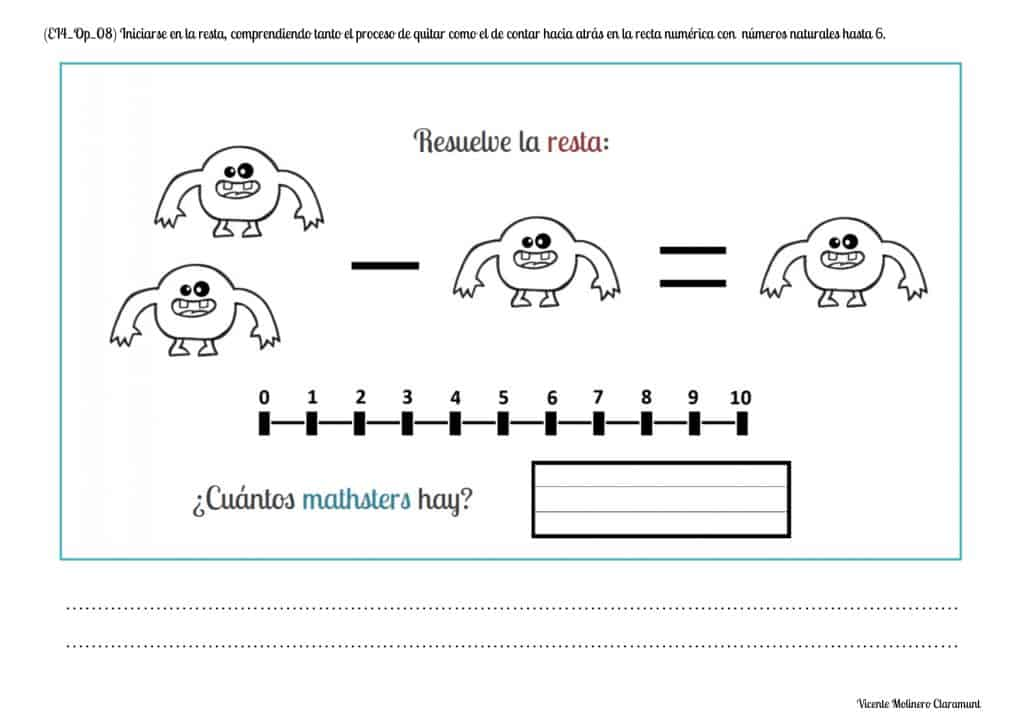#### Interpret the meaning of the subtraction signs (- e =) with natural numbers up to 6

PRE-KINDERGARTEN 4 YEARS

What do the symbols "-" and "=" mean in a subtraction?

From the 15th century, the current mathematical signs that represent the subtraction operation began to be used in Europe:

- The symbol "-" is read "minus", "less" or "subtracted from" and is always inserted between the elements that we have to subtract.

Example: The subtraction sentence 6 - 2 is read "six minus two"

- The symbol "=" is read "equals", "equal", "is" or "are" and represents the result that we obtain after reducing, separating or extracting the elements of a total set into a new set.

Example: The subtraction equation 6 - 2 = 4 is read "six minus two equals four"

SPANISHENGLISH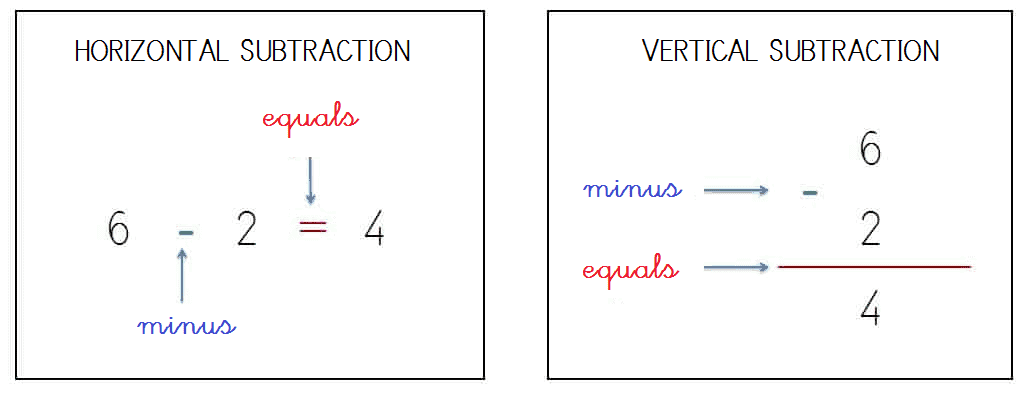We present you some files in PDF format where you have to count the elements of an initial collection and remove a part (also known as subtraction by crossing out) by demonstrating the equivalency between subtracting objects or subtracting numbers directly: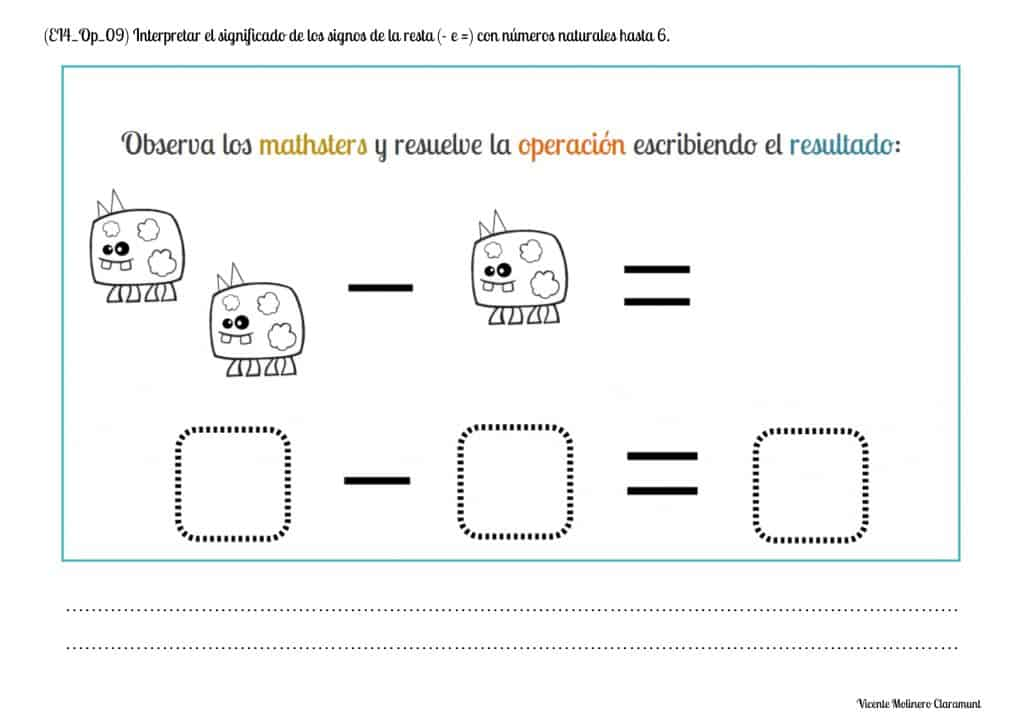#### Know the elements of subtraction and their position with natural numbers up to 6

PRE-KINDERGARTEN 4 YEARS

What is the "minuend", the "subtrahend" and the "difference" in a subtraction?

Once we have recognized and interpreted the meaning of the mathematical symbols "-" e "=", we will explain in detail what the subtraction terms are:

- Minuend: Represents the total collection or initial set of elements.

- Subtrahend: Symbolizes the number or quantity of elements in which the minuend will be reduced.

- Difference or Rest: It corresponds to the unique set formed by separating or reducing the amount of the subtrahend on the minuend.

SPANISHENGLISH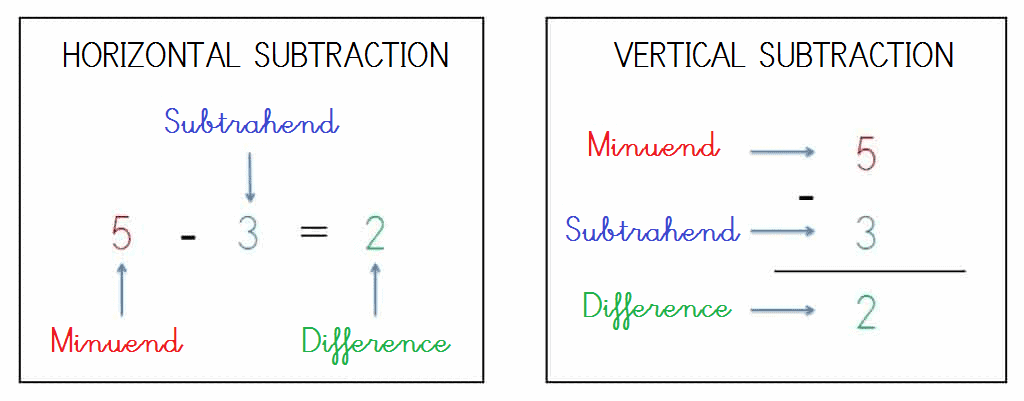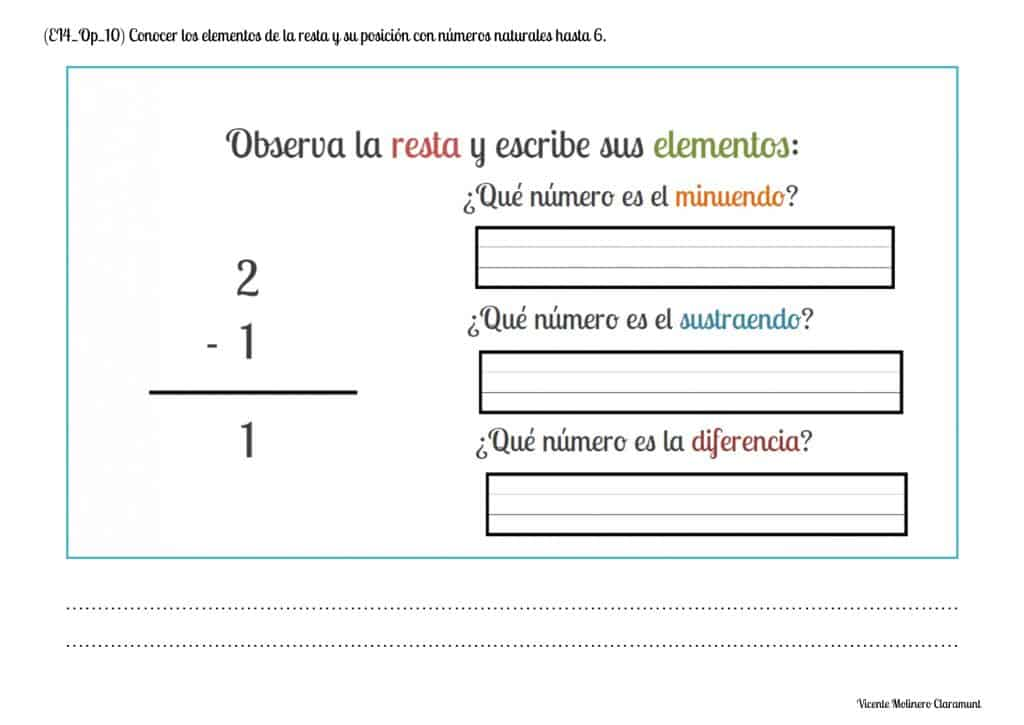#### Properly place the subtraction terms in natural numbers up to 6

PRE-KINDERGARTEN 4 YEARS

Next you will learn to construct vertical subtractions placing the symbols "-" e "=" and each of its terms (minuend, subtrahend and difference) in the corresponding place practicing with the different printable educational worksheets that we have produced.

SPANISHENGLISH#### Subtracting numbers vertically (with the help of the number line) with natural numbers up to 6

PRE-KINDERGARTEN 4 YEARS

Practice the subtraction without regrouping (or without borrowing) vertically (in columns) with the numbers from 1 to 6 using the number line from the exercises that we propose.

SPANISHENGLISH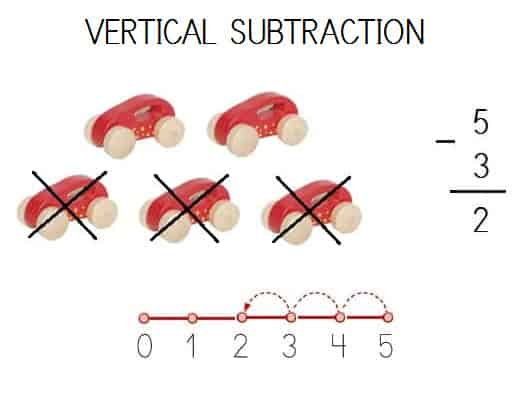#### Subtracting numbers vertically (without the help of the number line) with natural numbers up to 6

PRE-KINDERGARTEN 4 YEARS

We provide you with a series of exercises so that you can continue practicing subtraction no borrowing (or no regrouping) vertically with the numbers from 1 to 6... Remember that you can help with fingers!

SPANISHENGLISH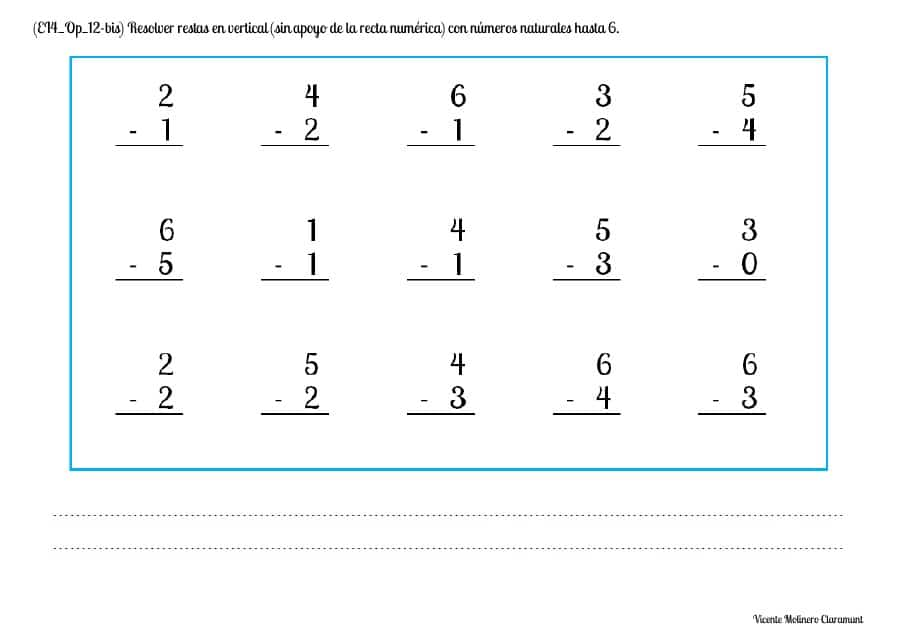#### Subtracting numbers horizontally (with the help of the number line) with natural numbers up to 6

PRE-KINDERGARTEN 4 YEARS

Keep practicing the subtraction without carrying horizontally with the numbers from 1 to 6 using the number line from our battery of PDF exercises.

SPANISHENGLISH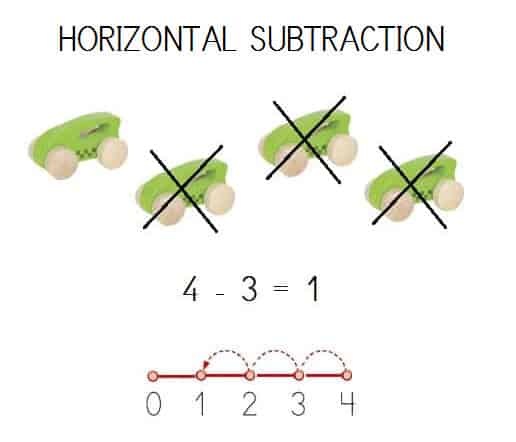#### Mentally solve simple subtraction facts with natural numbers up to 6

PRE-KINDERGARTEN 4 YEARS

Show your logical-mathematical skills by solving our exercises with hidden or missing whole numbers and using mental calculation (also known as "mental math").

SPANISHENGLISHLet's color!

We have selected dozens of Pinterest activities for you to practice coloring the basic addition and subtraction facts.

Open your case, take out the colored pencils and show the great artist in you!

Select more exercises, worksheets and activities of Mathematics for each of the 4 learning blocks of the Educational Curriculum ("Numbers and Operations", "Quantities and Measurements", "Geometric and Spatial Reasoning" and "Data Analysis and Probability") aimed at improving the logical-mathematical competencies and skills that are developed throughout "Pre-K4" or "Reception":

##### NUMBERS AND OPERATIONS
PRE-KINDERGARTEN 4 YEARS
##### QUANTITIES AND MEASUREMENTS
PRE-KINDERGARTEN 4 YEARS
##### GEOMETRIC AND SPATIAL REASONING
PRE-KINDERGARTEN 4 YEARS
##### DATA ANALYSIS AND PROBABILITY
PRE-KINDERGARTEN 4 YEARS
###### Data Collection and Organization

"You will always be a student, never a teacher"